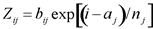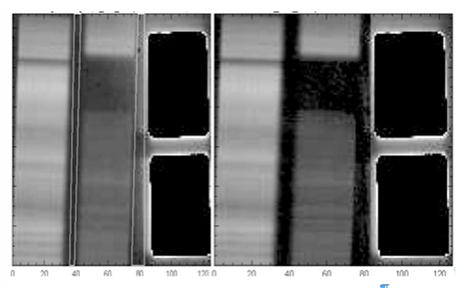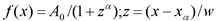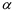Spitzer Documentation & Tools
IRS Instrument Handbook

### 5.1.16         REMOVSTRAYCROSS

The REMOVSTRAYCROSS module (“Remove Stray light or Crosstalk”) is comprised of two separate programs, depending on the IRS channel. The program SLREMOV corrects SL data for stray light falling onto the spectral orders from the peak-up subarrays. The program CROSSTALK corrects SH and LH data for interorder optical crosstalk, i.e., light leakage from one order to another. The programs assume a functional form for the light fall-off and subtract a fit to the data. REMOVSTRAYCROSS does not operate on LL data. The file rsc.fits is the output of REMOVSTRAYCROSS, with error plane rscu.fits.

#### 5.1.16.1   SLREMOV

The SL stray light signature is found to be well-described by an exponential decay from the edge of the peakup arrays leftward. The functionEquation 5.14

is fit to the data in row j in an interorder mask region. Here i is the column number, and constants aj (the normalization point) and nj (the decay length) describe the decaying behavior of the stray light along row j starting at the left edge of the peak-up sub-array. These {aj,nj} pairs are found to be relatively stable row-specific constants.

To improve signal to noise and ameliorate the effects of bad pixels, a median of five rows (rows j-2 to j+2) is used in the fit that is applied to row j. The stray light image Zij is built from the fits to these “combined” rows and subtracted from the data. In the event that there is little useful stray light signal in a given row (the stray light fit derives a signature with maximum level smaller than a specified multiple of the noise RMS), no stray light correction is done for that row. The peak-up subarrays are not affected by stray light correction.Figure 5.8 Correcting the stray light. The frame on the left displays a SL ground-based laboratory image. The peak-ups are saturated and have been blacked out for maximum contrast. The white lines drawn on the image delineate the areas in the stray light mask used to determine the parameters bij. The frame on the right shows the same image, corrected for stray light.

#### 5.1.16.2   CROSSTALK

The IRS SH and LH modules provide the high-resolution complement to the low-resolution modules through the use of echelle gratings. For each of these modules, the orders are projected on to the detectors in such a way that the short-wavelength ends of the orders are closer together on the detector than the long wavelength ends. This leads to optical leakage or cross-talk between the orders which is greatest at the short-wavelength end. The final design of the optics and the detector geometry is a compromise between obtaining good spectral coverage and minimizing cross-talk. For the IRS, the effect of cross-talk is minimal because the orders are not severely overlapping. However, in cases where the illuminating source is blue and bright the cross-talk can be as large as 10-15% at the edges of the short-wavelength orders, although only 2-3% near the center. Since we want to be able to recover the total emission in these blue orders, we provide a module (CROSSTALK) which automatically corrects for this effect.

The basic assumption of the crosstalk correction algorithm is that the intensity distribution of each order in the cross-dispersion direction can be fit with a simple function similar to a Lorentzian (see below). Experiments with laboratory test data have found that such a function is a good approximation to within a few percent of the shape of the cross-dispersed signal and moreover can adapt its basic shape to various forms of input structure, from a nearly perfect point-spread-function, to an extended source which fills the slit. The two main shape parameters are determined by fitting to the order least contaminated by cross-talk (hereafter the “uncontaminated order” or the m=11 order), and the results of this fit are used to constrain subsequent fits to all the bluer orders simultaneously in the same horizontal row across the image. In some cases the m=11 order is not bright enough to allow a reliable determination of the fitting parameters, and in these cases pre-determined “preferred” parameters are used to constrain the fits to the various orders. Although there is an implied assumption that line-tilt is small, the largest effects of crosstalk occur in the orders which are close together, where the assumption of zero line-tilt is almost valid.

The functional form found to best represent the shape of the uncontaminated order (based on ground-test data) is of the form:Equation 5.15

Here A0 is the maximum amplitude of the order at x=x02w, 2w is the FWHM of the order shape, andis the slope of the Lorentzian-like function. Indeed when=2 the function f(x) is exactly Lorentzian. We found for a wide range of fits to various uncontaminated orders, thatranges from 3 to 5 depending on the source.

The algorithm depends upon getting a good measurement of the shape-parameter for the reddest uncontaminated order (m=11). The FWHM of each order shape is scaled according to wavelength. Based on an initial pass through the input  data, the algorithm will decide to apply the crosstalk correction to the whole array, or to just the places where the emission is strong enough to justify correction. In some cases no correction will be made if the astronomical source is weak.